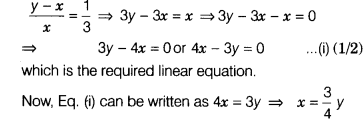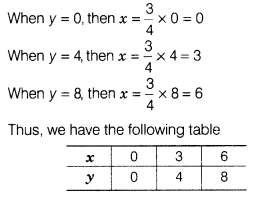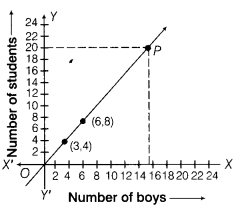# The ratio of girls and boys in a class is 1 : 3. Set-up an equation between the students of a class

The ratio of girls and boys in a class is 1 : 3. Set-up an equation between the students of a class and boys and then draw its graph. Also, find the number of boys in a class of 20 students from the graph.

Let the number of boys be x and the number of students be y.
∴ Number of girls = (y - x)
According to the question,Now, draw the graph by plotting the points (0, 0), (3, 4) and (6, 8) and join them by a line to get required graph.Now, draw a line parallel to X-axis from a point on Y-axis at a distance y =20, which intersects the graph at P. Now, draw perpendicular from P on X-axis, which intersects the X-axis at x = 15.

Hence, the required number of boys is 15.